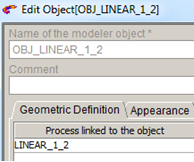# Linear repetition of objects

## Introduction

The operation Linear repetition of objects allows for the duplication of N times the objects according to a vector.

## Structure of the operation Linear repetition

The operation Linear repetition of the objects is described by:

• A coordinate system for the creation of points (active coordinate system in the list available on the graphic View)
• A list of objects to repeat
• The components on Ox, Oy and Oz of the vector of repetition
• The number of repetitions
• The appearance of the resulting objects (color and visibility)
Dialog box Illustration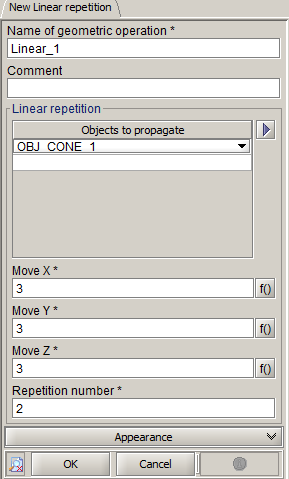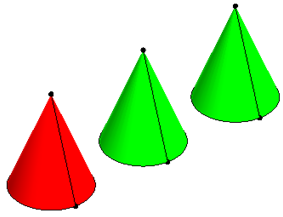## Result

The creation of an operation of Linear repetition has as results:

• A geometric operation LINEAR_1.
• As many geometric operations as the objects repeated LINEAR_1_1, LINEAR_1_2… (it permits to isolate each object duplicated and to use them independently then).
• As many objects as the objects to turn symmetric OBJ_ LINEAR _1_1, OBJ_ LINEAR _1_2, …
• The addition of the geometric operation LINEAR _1 in the list of geometric operations of each of origin objects
• The addition of the geometric operations LINEAR_1_1, LINEAR_1_2… in each of the objects resulting from the repetition (OBJ_LINEAR _1_1, OBJ_LINEAR _1_2, …)

## Access

The different ways to access the operation of Linear repetition are:

• By menu: Tools > Linear repetition > New
• By icon: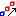## Creation of a linear repetition

The creation process of a linear repetition is presented in the table below.

Stage Description
1 Choice of the coordinate system of creation
2 Opening the dialog box Linear repetition
3

Choice of the name of the generated Geometric operation

(by default: LINEAR_1)

4 Choice of objects to repeat
5 Enter the coordinates of repetition vector (DX, DY, DZ)
6 Enter the number of repetitions
7 Validation by clicking on OK

## Limitations

In the modification only the vector of repetition and the number of repetitions are modifiable.

The list of repeated objects is not modifiable.

## Example of linear repetition

By means of an example, here are the geometric operations and the objects that are created during an operation of linear repetition of objects.

Studied example: repetition of a cone (number of repetitions = 2)

Before repetition After repetition
Data Tree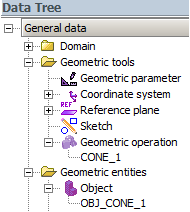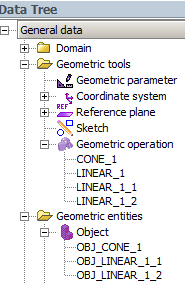Objects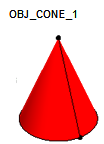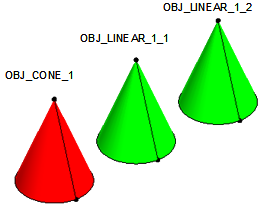Geometric operations

1 geometric operation:

• CONE_1: creation of the object OBJ_CONE_1

3 new geometric operations:

• (Father) LINEAR_1: creation of the repetition
• (Son) LINEAR_1_1: creation of the object OBJ_LINEAR_1_1
• (Son) LINEAR_1_2: creation of the object OBJ_LINEAR_1_2
Building tree of the object cone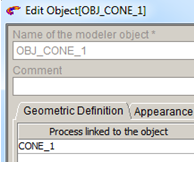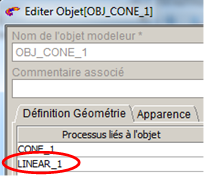Building trees of the resulting objects _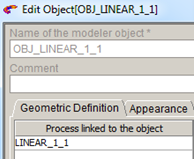_